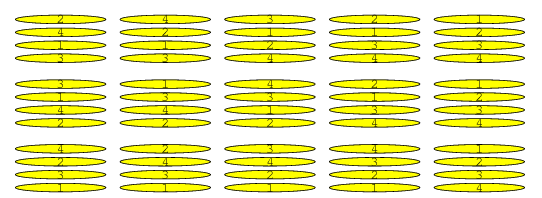# Pancake Sort Basic Information

suggest change

Pancake Sort is a the colloquial term for the mathematical problem of sorting a disordered stack of pancakes in order of size when a spatula can be inserted at any point in the stack and used to flip all pancakes above it. A pancake number is the minimum number of flips required for a given number of pancakes.

Unlike a traditional sorting algorithm, which attempts to sort with the fewest comparisons possible, the goal is to sort the sequence in as few reversals as possible.

The idea is to do something similar to Selection Sort. We one by one place maximum element at the end and reduce the size of current array by one.

Dissecting the problem:

1. Need to order the pancakes from smallest (top) to largest (bottom), the starting stack can be arranged in any order.
2. I only can perform flip flipping the entire stack.
3. To flip a specific pancake to the bottom of the stack, we first must flip it to the top (then flip it again to the bottom).
4. To order each pancake will require one flip up to the top and one flip down to its final location.

Intuitive Algorithm:

1. Find the largest out of order pancake and flip it to the bottom (you may need to flip it to the top of the stack first).
2. Repeat step one until the stack is ordered.
3. That’s it, a two step algorithm will work.

Example of Pancake sort algorithm:Auxiliary Space: `O(1)`

Time Complexity: `O(n^2)`